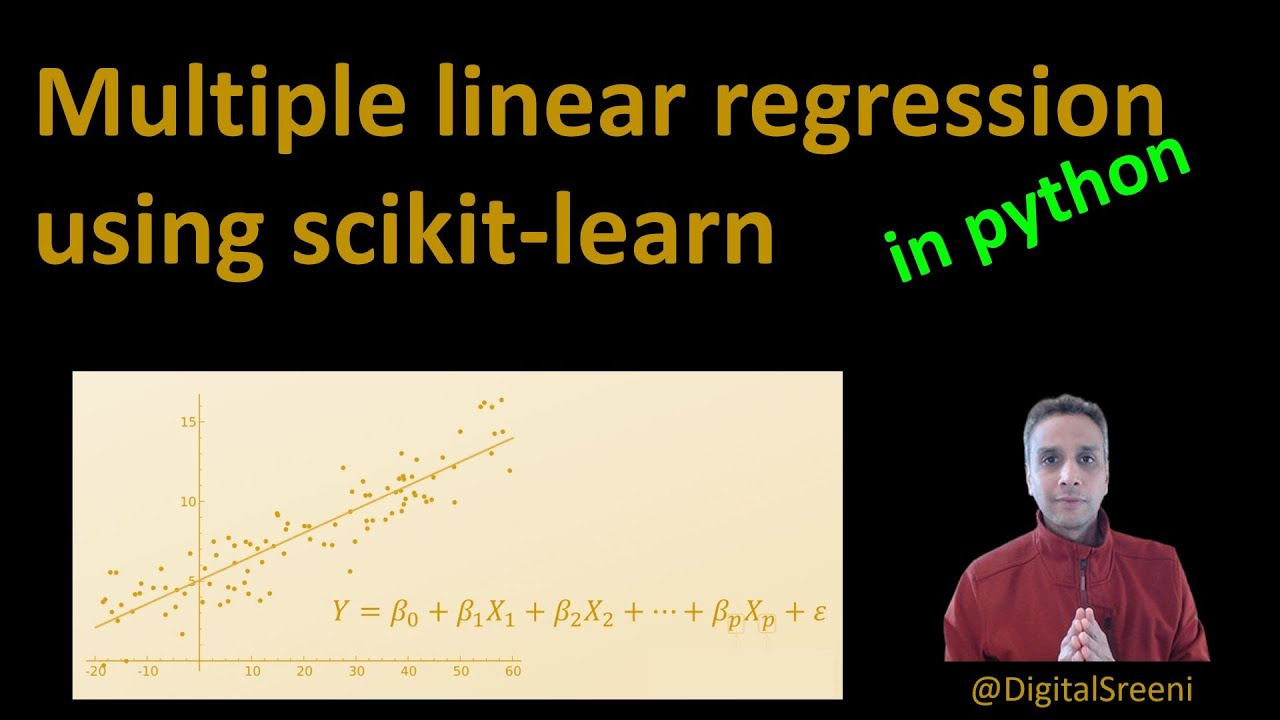1609147209

# Multiple Linear Regression with SciKit-Learn in Python

In the previous video I’ve explained the concept of linear regression where a single independent variable (X) was used to model the dependent variable (Y). In reality, multiple independent factors (variables) may influence the response. This tutorial explains the use of regression to model single independent variable using multiple dependent variables.

The code from this video is available at: https://github.com/bnsreenu/python_for_microscopists

#python #scikit